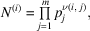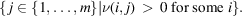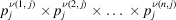International
Tables for
Crystallography
Volume B
Reciprocal space
Edited by U. Shmueli

International Tables for Crystallography (2010). Vol. B, ch. 1.3, pp. 59-60   | 1 | 2 |

## Section 1.3.3.3.2.2. Multidimensional prime factor algorithm

G. Bricognea

aGlobal Phasing Ltd, Sheraton House, Suites 14–16, Castle Park, Cambridge CB3 0AX, England, and LURE, Bâtiment 209D, Université Paris-Sud, 91405 Orsay, France

#### 1.3.3.3.2.2. Multidimensional prime factor algorithm

| top | pdf |

Suppose that the decimation matrix N is diagonaland let each diagonal element be written in terms of its prime factors:where m is the total number of distinct prime factors present in the.

The CRT may be used to turn each 1D transform along dimension iinto a multidimensional transform with a separate pseudo-dimension' for each distinct prime factor of; the number, of these pseudo-dimensions is equal to the cardinality of the set:The full n-dimensional transform thus becomes μ-dimensional, with.

We may now permute the μ pseudo-dimensions so as to bring into contiguous position those corresponding to the same prime factor; the m resulting groups of pseudo-dimensions are said to define p-primary' blocks. The initial transform is now written as a tensor product of m p-primary transforms, where transform j is onpoints [by convention, dimension i is not transformed if]. These p-primary transforms may be computed, for instance, by multidimensional Cooley–Tukey factorization (Section 1.3.3.3.1), which is faster than the straightforward row–column method. The final results may then be obtained by reversing all the permutations used.

The extra gain with respect to the multidimensional Cooley–Tukey method is that there are no twiddle factors between p-primary pieces corresponding to different primes p.

The case where N is not diagonal has been examined by Guessoum & Mersereau (1986).

### References

Guessoum, A. & Mersereau, R. M. (1986). Fast algorithms for the multidimensional discrete Fourier transform. IEEE Trans. Acoust. Speech Signal Process. 34, 937–943.